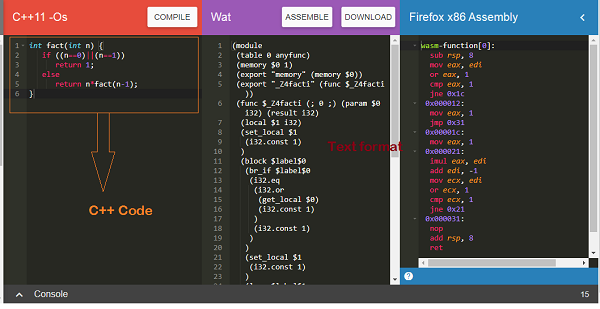# WebAssembly - Working with Nodejs

Javascript has a bunch of API that can work with wasm code. The API is also supported in nodejs.

Get NODEJS installed on your system. Create a Factorialtest.js file.

Let us use the C++ Factorial code as shown below −

```int fact(int n) {
if ((n==0)||(n==1))
return 1;
else
return n*fact(n-1);
}
```

Open Wasm Explorer, which is available at https://mbebenita.github.io/WasmExplorer/ as shown below −The first column has the C++ factorial function, the 2nd column has the WebAssembly text format and the last column has x86 Assembly code.

The WebAssembly Text format is as follows −

```(module
(table 0 anyfunc)
(memory \$0 1)
(export "memory" (memory \$0))
(export "_Z4facti" (func \$_Z4facti))
(func \$_Z4facti (; 0 ;) (param \$0 i32) (result i32)
(local \$1 i32)
(set_local \$1(i32.const 1))
(block \$label\$0
(br_if \$label\$0
(i32.eq
(i32.or
(get_local \$0)
(i32.const 1)
)
(i32.const 1)
)
)
(set_local \$1
(i32.const 1)
)
(loop \$label\$1
(set_local \$1
(i32.mul
(get_local \$0)
(get_local \$1)
)
)
(br_if \$label\$1
(i32.ne
(i32.or
(tee_local \$0
(get_local \$0)
(i32.const -1)
)
)
(i32.const 1)
)
(i32.const 1)
)
)
)
)
(get_local \$1)
)
)
```

The C++ function fact has been exported as “_Z4facti” in WebAssembly Text format.

Factorialtest.js

```const fs = require('fs');
const lib = WebAssembly.instantiate(new Uint8Array(buf)).
then(res => {
for (var i=1;i<=10;i++) {
console.log("The factorial of "+i+" = "+res.instance.exports._Z4facti(i))
}
}
);
```

In your command line, run the command node factorialtest.js and the output is as follows −

```C:\wasmnode>node factorialtest.js
The factorial of 1 = 1
The factorial of 2 = 2
The factorial of 3 = 6
The factorial of 4 = 24
The factorial of 5 = 120
The factorial of 6 = 720
The factorial of 7 = 5040
The factorial of 8 = 40320
The factorial of 9 = 362880
The factorial of 10 = 3628800
```